# HSSlive: Plus One & Plus Two Notes & Solutions for Kerala State Board

## AP Board Class 7 Maths Chapter 8 Congruency of Triangles Ex 2 Textbook Solutions PDF: Download Andhra Pradesh Board STD 7th Maths Chapter 8 Congruency of Triangles Ex 2 Book AnswersAP Board Class 7 Maths Chapter 8 Congruency of Triangles Ex 2 Textbook Solutions PDF: Download Andhra Pradesh Board STD 7th Maths Chapter 8 Congruency of Triangles Ex 2 Book Answers

## Andhra Pradesh State Board Class 7th Maths Chapter 8 Congruency of Triangles Ex 2 Books Solutions

 Board AP Board Materials Textbook Solutions/Guide Format DOC/PDF Class 7th Subject Maths Chapters Maths Chapter 8 Congruency of Triangles Ex 2 Provider Hsslive

2. Click on the Andhra Pradesh Board Class 7th Maths Chapter 8 Congruency of Triangles Ex 2 Answers.
3. Look for your Andhra Pradesh Board STD 7th Maths Chapter 8 Congruency of Triangles Ex 2 Textbooks PDF.
4. Now download or read the Andhra Pradesh Board Class 7th Maths Chapter 8 Congruency of Triangles Ex 2 Textbook Solutions for PDF Free.

## AP Board Class 7th Maths Chapter 8 Congruency of Triangles Ex 2 Textbooks Solutions with Answer PDF Download

Find below the list of all AP Board Class 7th Maths Chapter 8 Congruency of Triangles Ex 2 Textbook Solutions for PDF’s for you to download and prepare for the upcoming exams:

Question 1.
What additional information do you need to conclude that the two triangles given here under are congruent using SAS rule?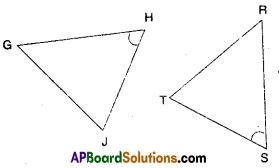Solution:
We need
HG = TS
HJ = TR

Question 2.
The map given below shows five different villages. Village M lies exactly halfway between the two pairs of villages A and B as well as and P and Q. What is the distance between village A and village P. (Hint: check if ΔPAM ≅ ΔQBM)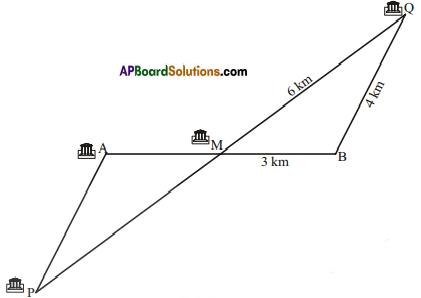Solution:
Given that
AM = MB
PM = MQ
also ∠PMA = ∠QMB
∴ By S.A.S
ΔPMA ≅ ΔQMB
Now PA = BQ
(∵ Corresponding parts of congruent triangles are equal)
∴ PA = 4 km
i.e., Distance between the villages
A and P is 4 km.

Question 3.
Look at the pairs of triangles given below. Are they congruent ? If congruent write the corresponding parts.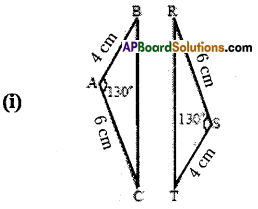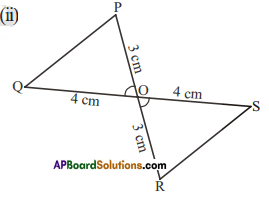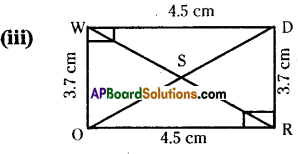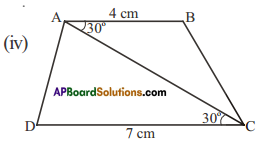Solution:AB=ST
AC = SR (given)
∠A = ∠S
∴ ΔABC ≅ ΔSTR (S.A.S)
Also ∠A = ∠S, ∠B = ∠T, ∠C = ∠RFrom the figure,
FO = RO
OQ = OS
∠POQ = ∠ROS
∴ ΔPOQ ≅ ΔROS (SAS)
Also ∠P = ∠R, ∠Q = ∠S, and PQ = RSFrom the figure, IAPBoardSoIJ
WD = OR
∠W= ∠R
WO = RD
∴ ΔDWO ≅ ΔORD
Also ∠EO = ∠OE; ∠WDO = ∠ROD; ∠WOD = ∠RDOHere the two triangles ΔABC and ΔCDA are not congruent.

Question 4.
Which corresponding sides do we need to know to prove that the triangles are congruent using the SAS criterion?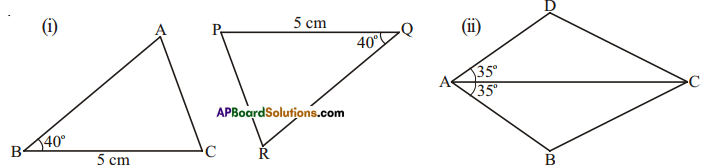Solution:
i) We need to know that AB = QR.
ii) We need to know that AD = AB.

## Andhra Pradesh Board Class 7th Maths Chapter 8 Congruency of Triangles Ex 2 Textbooks for Exam Preparations

Andhra Pradesh Board Class 7th Maths Chapter 8 Congruency of Triangles Ex 2 Textbook Solutions can be of great help in your Andhra Pradesh Board Class 7th Maths Chapter 8 Congruency of Triangles Ex 2 exam preparation. The AP Board STD 7th Maths Chapter 8 Congruency of Triangles Ex 2 Textbooks study material, used with the English medium textbooks, can help you complete the entire Class 7th Maths Chapter 8 Congruency of Triangles Ex 2 Books State Board syllabus with maximum efficiency.

## FAQs Regarding Andhra Pradesh Board Class 7th Maths Chapter 8 Congruency of Triangles Ex 2 Textbook Solutions

#### Can we get a Andhra Pradesh State Board Book PDF for all Classes?

Yes you can get Andhra Pradesh Board Text Book PDF for all classes using the links provided in the above article.

## Important Terms

Andhra Pradesh Board Class 7th Maths Chapter 8 Congruency of Triangles Ex 2, AP Board Class 7th Maths Chapter 8 Congruency of Triangles Ex 2 Textbooks, Andhra Pradesh State Board Class 7th Maths Chapter 8 Congruency of Triangles Ex 2, Andhra Pradesh State Board Class 7th Maths Chapter 8 Congruency of Triangles Ex 2 Textbook solutions, AP Board Class 7th Maths Chapter 8 Congruency of Triangles Ex 2 Textbooks Solutions, Andhra Pradesh Board STD 7th Maths Chapter 8 Congruency of Triangles Ex 2, AP Board STD 7th Maths Chapter 8 Congruency of Triangles Ex 2 Textbooks, Andhra Pradesh State Board STD 7th Maths Chapter 8 Congruency of Triangles Ex 2, Andhra Pradesh State Board STD 7th Maths Chapter 8 Congruency of Triangles Ex 2 Textbook solutions, AP Board STD 7th Maths Chapter 8 Congruency of Triangles Ex 2 Textbooks Solutions,
Share: